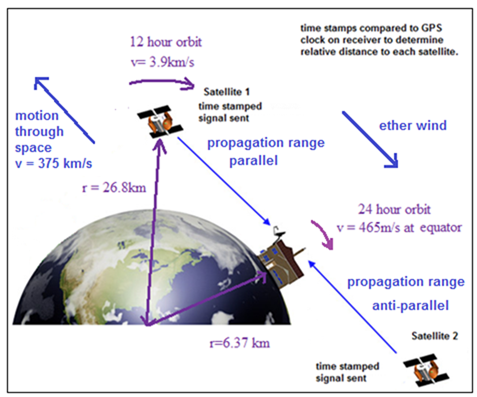﻿ GPSsimulator
 GPS Propagation Range SimulatorPropagation range (Km) Wavelength on Each Path: velocity through space (m/s) Freq of xmtr (Hz) l  C4 path with wind l  C4 path against wind Vrot satellite (m/s) radius of rotation (Km): Velocities on Each Path: earth rot. V at latitude (m/s) V  C4 Path with wind V  C4 Path against wind wavelength of xmtr (m) Path Effect (satellite to earth) Clock Effect on satellite: Satellite Satellite Propagation Time for 180 deg. rotation (s): Clock Effect Time Delay (sec) parallel to ether wind: anti-parallel to wind Time Difference (sec) t*(1/(1-v^2/c^2)*(L/2c^2)*V*wrot*sin(q) Propagation Time (s) Propagation Time (s) wrot Laser: Sagnac Effect Error (when uncorrected) Error due to velocity through space (negligible) Dt Sagnac effect (sec): Range Error (m) Dt Path Effect - Clock effect (sec): Propagation Range Error (m) Why is our translational motion through space never detected in GPS satellite range tests? This simulation attempts to explain why GPS transmissions do not detect our translational motion through space. As would be predicted by Lorentz ether theory (1904) the transmitting clock undergoes an additive time dilation as it orbits the earth which depends on the product of the translational velocity through space and the spin velocity. This alters the synchronization between the transmitting clock and the receiving clock, by exactly the amount to cancel any propagation range time difference due to the one-way speed of light in a particular direction. Since this time dilation is never corrected in GPS satellites, the net result is that the measured speed of light along the one way path is always equal to C. However, the much smaller rotational velocity of the earth (Sagnac effect) is readily detected and must be corrected for, otherwise a large range error occurs. In this simulation, we compare the propagation time for a signal assuming a net motion past the Cosmic Micromave Background Radiation (CMBr) as a preferred frame for the speed of light, consistent with the original thinking of Lorentz. Although a large time difference due to our motion should occur, it is cancelled out by the change in the clock speed of the transmitter.  As a consequence, Lorentz ether theory and Special Relativity predict the same outcome, namely a measured speed of light of C. Adjustable parameters are in the yellow boxes, including the various velocities and ranges.